# CLASS 11 MATHS CHAPTER-6 LINEAR INEQUALITIES

### Exercise 6.1

Question1. Solve 24x < 100, when (i) x is a natural number (ii) x is an integer

Solution :
The given inequality is 24x < 100.

Dividing both sides by 24,

⇒ 24x / 24  <  100 / 24

⇒x  < 25/6

(i) It is evident that 1, 2, 3, and 4 are the only natural numbers less than 25/6.

Thus, when x is a natural number, the solutions of the given inequality are 1, 2, 3, and 4.

Hence, in this case, the solution set is {1, 2, 3, 4}.

(ii) The integers less than 25/6 are …–3, –2, –1, 0, 1, 2, 3, 4.

Thus, when x is an integer, the solutions of the given inequality are

…–3, –2, –1, 0, 1, 2, 3, 4.

Hence, in this case, the solution set is {…–3, –2, –1, 0, 1, 2, 3, 4}.

Question2. Solve –12x > 30, when

(i) x is a natural number

(ii) x is an integer

Solution :
Given:  −12x > 30.

Dividing both sides by ⇒ −12x  / −12 < 30 / −12

(Dividing both sides by same negative number)

⇒ x<−5 / 2.

(i) When x is a natural number then values of x that make statement true are none.

(ii) When x is an integer then values of x that make statement true are …, –5, –4, –3. The solution set of inequality is …, –5, –4, –3.

Question3. Solve 5x– 3 < 7, when

(i) x is an integer (ii) x is a real number

Solution :
The given inequality is 5x– 3 < 7

(i) The integers less than 2 are …, –4, –3, –2, –1, 0, 1.

Thus, when x is an integer, the solutions of the given inequality are

…, –4, –3, –2, –1, 0, 1.

Hence, in this case, the solution set is {…, –4, –3, –2, –1, 0, 1}.

(ii) When x is a real number, the solutions of the given inequality are given by x < 2, that is, all real numbers x which are less than 2.

Thus, the solution set of the given inequality is x ∈ (–∞, 2).

Question 4 . Solve 3x + 8 > 2, when

(i) x is an integer

(ii) x is a real number

Solution :
The given inequality is 3x + 8 > 2.

⇒ 3x + 8 – 8 > 2 – 8

⇒  3x > – 6

⇒  3x/ 3  >  – 6/3
⇒ x  -2

(i) The integers greater than –2 are –1, 0, 1, 2, …

Thus, when x is an integer, the solutions of the given inequality are

–1, 0, 1, 2 …

Hence, in this case, the solution set is {–1, 0, 1, 2, …}.

(ii) When x is a real number, the solutions of the given inequality are all the real numbers, which are greater than –2.

Thus, in this case, the solution set is (– 2, ∞).

Question5. Solve the given inequality for real x: 4x + 3 < 5x + 7

Solution :
4x + 3 < 5x + 7

⇒ 4x + 3 – 7 < 5x + 7 – 7

⇒ 4x – 4 < 5x

⇒ 4x – 4 – 4x < 5x – 4x

⇒ –4 < x

Thus, all real numbers x,which are greater than –4, are the solutions of the given inequality.

Hence, the solution set of the given inequality is (–4, ∞).

Question6. Solve the given inequality for real x: 3x – 7 > 5x – 1

Solution :
3x – 7 > 5x – 1

⇒ 3x – 7 + 7 > 5x – 1 + 7

⇒ 3x > 5x + 6

⇒ 3x – 5x > 5x + 6 – 5x

⇒ – 2x > 6

⇒ – 2x / -2 <  6 / 2

⇒ x  < -3

Therefore, the solution set is x

Question7. Solve the given inequality for real x: 3(x – 1) ≤ 2 (x – 3)

Solution :
3(x – 1) ≤ 2(x – 3)

⇒ 3x – 3 ≤ 2x – 6

⇒ 3x – 3 + 3 ≤ 2x – 6 + 3

⇒ 3x ≤ 2x – 3

⇒ 3x – 2x ≤ 2x – 3 – 2x

⇒ x ≤ – 3

Thus, all real numbers x,which are less than or equal to –3, are the solutions of the given inequality.

Hence, the solution set of the given inequality is (–∞, –3].

Question8. Solve the given inequality for real x: 3 (2 – x) ≥ 2 (1 – x)

Solution :
3(2 – x) ≥ 2(1 – x)

⇒ 6 – 3x ≥ 2 – 2x

⇒ 6 – 3x + 2x ≥ 2 – 2x + 2x

⇒ 6 – x ≥ 2

⇒ 6 – x – 6 ≥ 2 – 6

⇒ –x ≥ –4

⇒ x ≤ 4

Thus, all real numbers x,which are less than or equal to 4, are the solutions of the given inequality.

Hence, the solution set of the given inequality is (–∞, 4].

Question9: Solve the given inequality for real x:

Solution :
Here

Question10.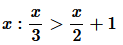Solution :
HereQuestion11.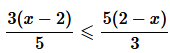Solution :

given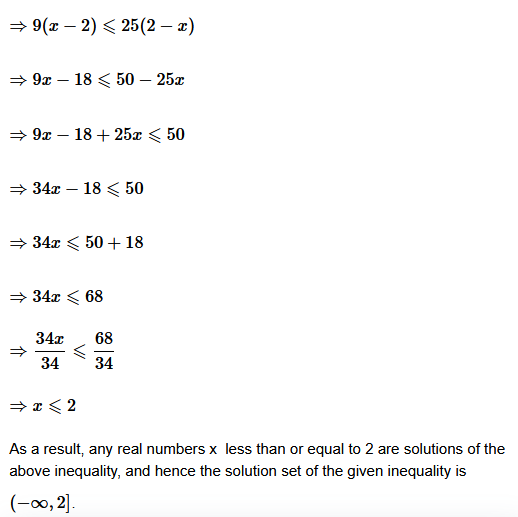Question12.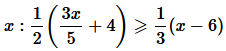Solution :
Here

Question13. Solve the given inequality for real x: 2(2x + 3) – 10 < 6 (x – 2)

Solution :⇒4x+6−10<6x−12

⇒4x−4<6x−12

⇒−4+12<6x−4x

⇒8<2x

⇒4<x

As a result, any real numbers  x  bigger than 4 are solutions to the specified inequality. As a result, the given inequality’s solution set is  (4,−∞)
.

Question14. Solve the given inequality for real x: 37 – (3x + 5)  ≥ 9x –  8(x – 3)

Solution :
Here

⇒37−3x−5⩾9x−8x+24

⇒32−3x⩾x+24

⇒32−24⩾x+3x

⇒8⩾4x

⇒2⩾x

As a result, the solutions of the following inequality are all real values  x less than or equal to 2. As a result, the given inequality’s solution set is  (−∞,2]

Question15.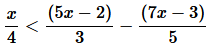Solution :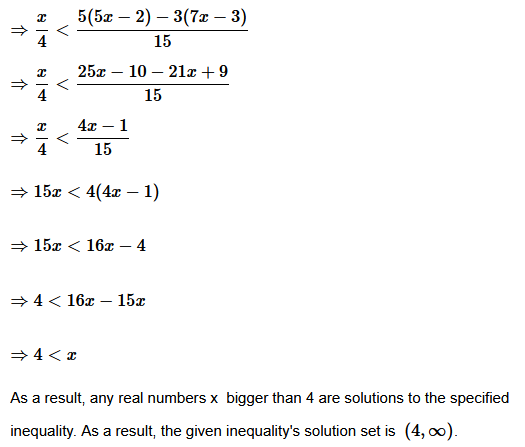Question16.Solution :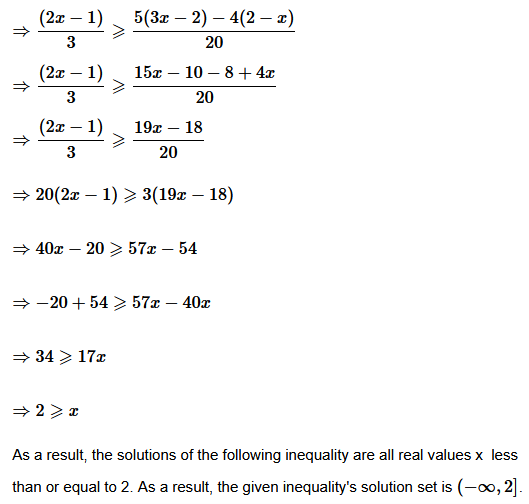Solve the inequalities in Exercises 17 to 20 and show the graph of the solution in each case on number line:

Question17. Solve the given inequality and show the graph of the solution on number line: 3x – 2 < 2x +1

Solution :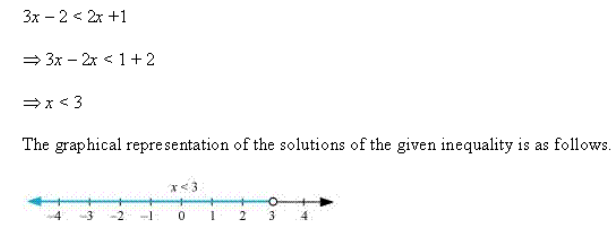Question18. Solve the given inequality and show the graph of the solution on number line: 5x – 3 ≥ 3x – 5

Solution :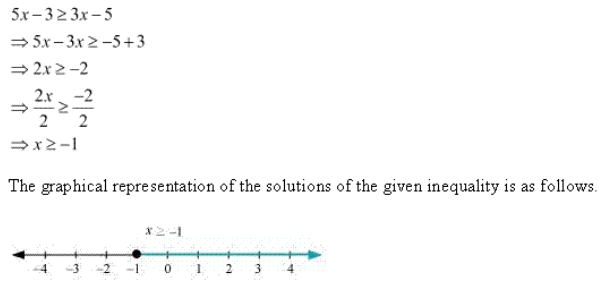Question19. Solve the given inequality and show the graph of the solution on number line: 3(1 – x) < 2 (x + 4)

Solution :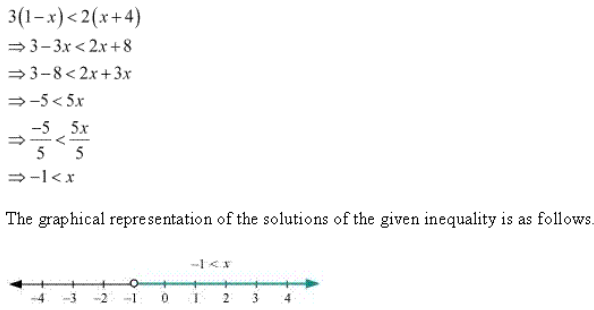Question20. Solve the given inequality and show the graph of the solution on number line: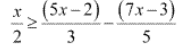Solution :
Here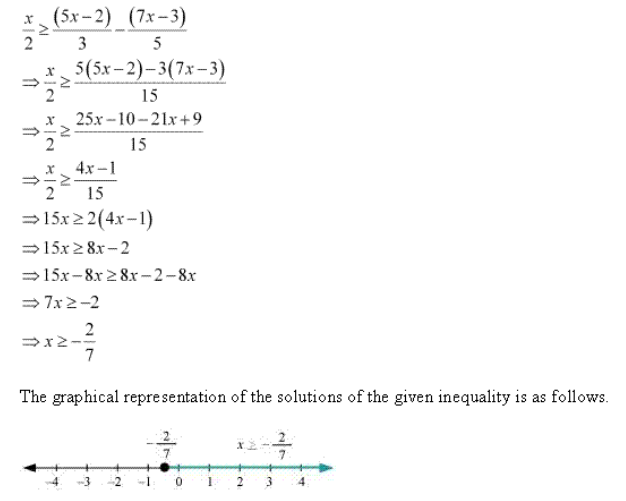Question21. Ravi obtained 70 and 75 marks in first two unit tests. Find the minimum marks he should get in the third test to have an average of at least 60 marks.

Solution :
Let the marks obtained by Ravi in third test be x.

According to question,

⇒145+x⩾180

⇒x⩾180−145

⇒x⩾35

As a result, the student must score at least 35 points to achieve a 60-point average.

Question22. To receive Grade ‘A’ in a course, one must obtain an average 90 marks or more in five examinations (each of 100 marks). If Sunita’s marks in first four examinations are 87, 92, 94 and 95, find minimum marks that Sunita must obtain in fifth examination to get Grade ‘A’ in the course.

Solution :Let x represent Sunita’s grade in the fifth examination. She must receive an average of 90 or above in five examinations in order to receive a grade of  in the course,

Question23. Find all pairs of consecutive odd positive integers both of which are smaller than 10 such that their sum is more than 11.

Solution :
Let x and x + 2 be two consecutive odd positive integers.

Let x be the lesser of the two odd positive integers that follow. Then there’s x + 2 as the other integer. Because both integers are less than  10 , x+2<10

⇒x<10−2

⇒x<8….(t)

Furthermore, the sum of the two integers exceeds 11.∴x+(x+2)>11

⇒2x+2>11

⇒2x>11−2

⇒2x>9

⇒x>9/2

⇒x>4.5

We get I and (ii) from I and (ii).

Because x is an odd number, the values 5 and 7 can be used.

As a result, the required pairs are (5,7)
and (7,9).

Therefore, the required pairs of odd positive integers are (5, 7) and (7, 9).

Question24. Find all pairs of consecutive even positive integers, both of which are larger than 5 such that their sum is less than 23.

Solution : Let be the smaller of the two even positive integers that follow. The other integer is x + 2

because both integers are greater than 5, x>5……(1)

Also, the sum of the two integers is less than 23 x+(x+2)<23

⇒2x+2<23

⇒2x<23−2

⇒2x<21

⇒x<21 / 2

⇒x<10.5……(2)

From (1)
and (2), we obtain 5<x<10.5

Because x is an even number, it can have any of the following values: 6,8, or 10.

As a result, the required pairs are (6,8),(8,10)
and (10,12).

Therefore, the required pairs of even positive integers are (6,8), (8 10) and (10, 12).

Question25. The longest side of a triangle s 3 times the shortest side and the third side is 2 cm shorter than the longest side. If the perimeter of the triangle is at least 61 cm. Find the minimum length of the shortest side.

Solution :Determine the length of the triangle’s shortest side  xcm. Then, length of the longest side =3xcm Length of the third side (3x−2)cm Since the perimeter of the triangle is at least 61cm, xcm+3xcm+(3x−2)cm⩾61cm

⇒7x−2⩾61

⇒7x⩾61+2

⇒7x⩾63

⇒7x / 7⩾63 / 7

⇒x⩾9

As a result, the shortest side’s minimal length is  9cm
.

Question26. A man wants to cut three lengths from a single piece of board of length 91 cm. The second length is to be 3 cm longer than the shortest and the third length is to be twice as long as the shortest. What are the possible lengths of the shortest board if the third piece is to be at least 5 cm longer than the second?

Solution :
The shortest piece’s length should be xcm. Then, length of the second piece and the third piece are (x+3)cm and 2xcm respectively. Since the three lengths are to be cut from a single piece of board of length 91cm,

x cm+(x+3) cm+2xcm⩽91cm

⇒4x+3⩽91

⇒4x⩽91−3

⇒4x⩽88

⇒4x / 4⩽88 / 4

⇒x⩽22….(1)

In addition, the third component is at least 5 cm as long as the second. ∴2x⩾(x+3)+5

⇒2x⩾x+8

⇒x⩾8

From (1) and (2), we obtain 8⩽x⩽22

As a result, the smallest board’s potential length is larger than or equal to but less than or equal to 22cm

### Solve the following inequalities graphically in two dimensional planes:

Question1. Solve the given inequality graphically in two-dimensional plane: x + y < 5

Solution :
The graphical representation of x + y = 5 is given as dotted line in the figure below.

This line divides the xy-plane in two half planes, I and II.

Select a point (not on the line), which lies in one of the half planes, to determine whether the point satisfies the given inequality or not.

We select the point as (0, 0).

It is observed that,

0 + 0 < 5 or, 0 < 5, which is true

Therefore, half plane II is not the solution region of the given inequality. Also, it is evident that any point on the line does not satisfy the given strict inequality.

Thus, the solution region of the given inequality is the shaded half plane I excluding the points on the line.

This can be represented as follows.

Question2. Solve the given inequality graphically in two-dimensional plane: 2x + y ≥ 6

Solution :
The graphical representation of 2x + y = 6 is given in the figure below.

This line divides the xy-plane in two half planes, I and II.

Select a point (not on the line), which lies in one of the half planes, to determine whether the point satisfies the given inequality or not.

We select the point as (0, 0).

It is observed that,

2(0) + 0 ≥ 6 or 0 ≥ 6, which is false

Therefore, half plane I is not the solution region of the given inequality. Also, it is evident that any point on the line satisfies the given inequality.

Thus, the solution region of the given inequality is the shaded half plane II including the points on the line.

This can be represented as follows.

Question3. Solve the given inequality graphically in two-dimensional plane: 3x + 4y ≤ 12

Solution :
3x + 4y ≤ 12

The graphical representation of 3x + 4y = 12 is given in the figure below.

This line divides the xy-plane in two half planes, I and II.

Select a point (not on the line), which lies in one of the half planes, to determine whether the point satisfies the given inequality or not.

We select the point as (0, 0).

It is observed that,

3(0) + 4(0) ≤ 12 or 0 ≤ 12, which is true

Therefore, half plane II is not the solution region of the given inequality. Also, it is evident that any point on the line satisfies the given inequality.

Thus, the solution region of the given inequality is the shaded half plane I including the points on the line.

This can be represented as follows.

Question4.Solve the given inequality graphically in two-dimensional plane: y + 8 ≥ 2x

Solution :
The graphical representation of y + 8 = 2x is given in the figure below.

This line divides the xy-plane in two half planes.

Select a point (not on the line), which lies in one of the half planes, to determine whether the point satisfies the given inequality or not.

We select the point as (0, 0).

It is observed that,

0 + 8 ≥ 2(0) or 8 ≥ 0, which is true

Therefore, lower half plane is not the solution region of the given inequality. Also, it is evident that any point on the line satisfies the given inequality.

Thus, the solution region of the given inequality is the half plane containing the point (0, 0) including the line.

The solution region is represented by the shaded region as follows.

Question5.Solve the given inequality graphically in two-dimensional plane: x – y ≤ 2

Solution :
The graphical representation of x – y = 2 is given in the figure below.

This line divides the xy-plane in two half planes.

Select a point (not on the line), which lies in one of the half planes, to determine whether the point satisfies the given inequality or not.

We select the point as (0, 0).

It is observed that,

0 – 0 ≤ 2 or 0 ≤ 2, which is true

Therefore, the lower half plane is not the solution region of the given inequality. Also, it is clear that any point on the line satisfies the given inequality.

Thus, the solution region of the given inequality is the half plane containing the point (0, 0) including the line.

The solution region is represented by the shaded region as follows.

Question6.Solve the given inequality graphically in two-dimensional plane: 2x – 3y > 6

Solution :
The graphical representation of 2x – 3y = 6 is given as dotted line in the figure below. This line divides the xy-plane in two half planes.

Select a point (not on the line), which lies in one of the half planes, to determine whether the point satisfies the given inequality or not.

We select the point as (0, 0).

It is observed that,

2(0) – 3(0) > 6 or 0 > 6, which is false

Therefore, the upper half plane is not the solution region of the given inequality. Also, it is clear that any point on the line does not satisfy the given inequality.

Thus, the solution region of the given inequality is the half plane that does not contain the point (0, 0) excluding the line.

The solution region is represented by the shaded region as follows.

Question7.Solve the given inequality graphically in two-dimensional plane: –3x + 2y ≥ –6

Solution :
The graphical representation of – 3x + 2y = – 6 is given in the figure below.

This line divides the xy-plane in two half planes.

Select a point (not on the line), which lies in one of the half planes, to determine whether the point satisfies the given inequality or not.

We select the point as (0, 0).

It is observed that,

– 3(0) + 2(0) ≥ – 6 or 0 ≥ –6, which is true

Therefore, the lower half plane is not the solution region of the given inequality. Also, it is evident that any point on the line satisfies the given inequality.

Thus, the solution region of the given inequality is the half plane containing the point (0, 0) including the line.

The solution region is represented by the shaded region as follows.

Question8.Solve the given inequality graphically in two-dimensional plane: 3y – 5x < 30

Solution :
The graphical representation of 3y – 5x = 30 is given as dotted line in the figure below.

This line divides the xy-plane in two half planes.

Select a point (not on the line), which lies in one of the half planes, to determine whether the point satisfies the given inequality or not.

We select the point as (0, 0).

It is observed that,

3(0) – 5(0) < 30 or 0 < 30, which is true

Therefore, the upper half plane is not the solution region of the given inequality. Also, it is evident that any point on the line does not satisfy the given inequality.

Thus, the solution region of the given inequality is the half plane containing the point (0, 0) excluding the line.

The solution region is represented by the shaded region as follows.

Question9.Solve the given inequality graphically in two-dimensional plane: y < –2

Solution :
The graphical representation of y = –2 is given as dotted line in the figure below. This line divides the xy-plane in two half planes.

Select a point (not on the line), which lies in one of the half planes, to determine whether the point satisfies the given inequality or not.

We select the point as (0, 0).

It is observed that,

0 < –2, which is false

Also, it is evident that any point on the line does not satisfy the given inequality.

Hence, every point below the line, y = –2 (excluding all the points on the line), determines the solution of the given inequality.

The solution region is represented by the shaded region as follows.

Question10.Solve the given inequality graphically in two-dimensional plane: x > –3

Solution :
The graphical representation of x = –3 is given as dotted line in the figure below. This line divides the xy-plane in two half planes.

Select a point (not on the line), which lies in one of the half planes, to determine whether the point satisfies the given inequality or not.

We select the point as (0, 0).

It is observed that,

0 > –3, which is true

Also, it is evident that any point on the line does not satisfy the given inequality.

Hence, every point on the right side of the line, x = –3 (excluding all the points on the line), determines the solution of the given inequality.

The solution region is represented by the shaded region as follow

### Solve the following systems of inequalities graphically:

Question 1. Solve the following system of inequalities graphically: x ≥ 3, y ≥ 2

Solution :
x ≥ 3 … (1)

y ≥ 2 … (2)

The graph of the lines, x = 3 and y = 2, are drawn in the figure below.

Inequality (1) represents the region on the right hand side of the line, x = 3 (including the line x = 3), and inequality (2) represents the region above the line, y = 2 (including the line y = 2).

Hence, the solution of the given system of linear inequalities is represented by the common shaded region including the points on the respective lines as follows.

Question2. Solve the following system of inequalities graphically: 3x + 2y ≤ 12, x ≥ 1, y ≥ 2

Solution :
3x + 2y ≤ 12 … (1)

x ≥ 1 … (2)

y ≥ 2 … (3)

The graphs of the lines, 3x + 2y = 12, x = 1, and y = 2, are drawn in the figure below

Inequality (1) represents the region below the line, 3x + 2y = 12 (including the line 3x + 2y = 12). Inequality (2) represents the region on the right side of the line, x = 1 (including the line x = 1). Inequality (3) represents the region above the line, y = 2 (including the line y = 2).

Hence, the solution of the given system of linear inequalities is represented by the common shaded region including the points on the respective lines as follows.

Question3. Solve the following system of inequalities graphically: 2x + y≥ 6, 3x + 4y ≤ 12

Solution :
2x + y≥ 6 … (1)

3x + 4y ≤ 12 … (2)

The graph of the lines, 2x + y= 6 and 3x + 4y = 12, are drawn in the figure below.

Inequality (1) represents the region above the line, 2x + y= 6 (including the line 2x + y= 6), and inequality (2) represents the region below the line, 3x + 4y =12 (including the line 3x + 4y =12).

Hence, the solution of the given system of linear inequalities is represented by the common shaded region including the points on the respective lines as follows.

Question4. Solve the following system of inequalities graphically: x + y≥ 4, 2x – y > 0

Solution :
x + y≥ 4 … (1)

2x – y > 0 … (2)

The graph of the lines, x + y = 4 and 2x – y = 0, are drawn in the figure below.

Inequality (1) represents the region above the line, x + y = 4 (including the line x + y = 4).

It is observed that (1, 0) satisfies the inequality, 2x – y > 0. [2(1) – 0 = 2 > 0]

Therefore, inequality (2) represents the half plane corresponding to the line, 2x – y = 0, containing the point (1, 0) [excluding the line 2x – y > 0].

Hence, the solution of the given system of linear inequalities is represented by the common shaded region including the points on line x + y = 4 and excluding the points on line 2x – y = 0 as follows.

Question5. Solve the following system of inequalities graphically: 2x – y > 1, x – 2y < –1

Solution :
2x – y > 1 … (1)

x – 2y < –1 … (2)

The graph of the lines, 2x – y = 1 and x – 2y = –1, are drawn in the figure below.

Inequality (1) represents the region below the line, 2x – y = 1 (excluding the line 2x – y = 1), and inequality (2) represents the region above the line, x – 2y = –1 (excluding the line x – 2y = –1).

Hence, the solution of the given system of linear inequalities is represented by the common shaded region excluding the points on the respective lines as follows.

Question6. Solve the following system of inequalities graphically: x + y ≤ 6, x + y ≥ 4

Solution :
x + y ≤ 6 … (1)

x + y ≥ 4 … (2)

The graph of the lines, x + y = 6 and x + y = 4, are drawn in the figure below.

Inequality (1) represents the region below the line, x + y = 6 (including the line x + y = 6), and inequality (2) represents the region above the line, x + y = 4 (including the line x + y = 4).

Hence, the solution of the given system of linear inequalities is represented by the common shaded region including the points on the respective lines as follows.

Question7. Solve the following system of inequalities graphically: 2x + y≥ 8, x + 2y ≥ 10

Solution :
2x + y= 8 … (1)

x + 2y = 10 … (2)

The graph of the lines, 2x + y= 8 and x + 2y = 10, are drawn in the figure below.

Inequality (1) represents the region above the line, 2x + y = 8, and inequality (2) represents the region above the line, x + 2y = 10.

Hence, the solution of the given system of linear inequalities is represented by the common shaded region including the points on the respective lines as follows.

Question8. Solve the following system of inequalities graphically: x + y ≤ 9, y > x, x ≥ 0

Solution :
x + y ≤ 9      … (1)

y > x             … (2)

x ≥ 0             … (3)

The graph of the lines, x + y= 9 and y = x, are drawn in the figure below.

Inequality (1) represents the region below the line, x + y = 9 (including the line x + y = 9).

It is observed that (0, 1) satisfies the inequality, y > x. [1 > 0]

Therefore, inequality (2) represents the half plane corresponding to the line, y = x, containing the point (0, 1) [excluding the line y = x].

Inequality (3) represents the region on the right hand side of the line, x = 0 or y-axis (including y-axis).

Hence, the solution of the given system of linear inequalities is represented by the common shaded region including the points on the lines, x + y = 9 and x = 0, and excluding the points on line y = x as follows.

Question9. Solve the following system of inequalities graphically: 5x + 4y ≤ 20, x ≥ 1, y ≥ 2

Solution :
5x + 4y ≤ 20 … (1)

x ≥ 1 … (2)

y ≥ 2 … (3)

The graph of the lines, 5x + 4y = 20, x = 1, and y = 2, are drawn in the figure below.

Inequality (1) represents the region below the line, 5x + 4y = 20 (including the line 5x + 4y = 20). Inequality (2) represents the region on the right hand side of the line, x = 1 (including the line x = 1). Inequality (3) represents the region above the line, y = 2 (including the line y = 2).

Hence, the solution of the given system of linear inequalities is represented by the common shaded region including the points on the respective lines as follows.

Question10. Solve the following system of inequalities graphically: 3x + 4y ≤ 60, x + 3y ≤ 30, x ≥ 0, y ≥ 0

Solution :
3x + 4y ≤ 60 … (1)

x + 3y ≤ 30 … (2)

The graph of the lines, 3x + 4y = 60 and x + 3y = 30, are drawn in the figure below.

Inequality (1) represents the region below the line, 3x + 4y = 60 (including the line 3x + 4y = 60), and inequality (2) represents the region below the line, x + 3y = 30 (including the line x + 3y = 30).

Since x ≥ 0 and y ≥ 0, every point in the common shaded region in the first quadrant including the points on the respective line and the axes represents the solution of the given system of linear inequalities.

Question11. Solve the following system of inequalities graphically: 2x + y≥ 4, x + y ≤ 3, 2x – 3y ≤ 6

Solution :
2x + y≥ 4 … (1)

x + y ≤ 3 … (2)

2x – 3y ≤ 6 … (3)

The graph of the lines, 2x + y= 4, x + y = 3, and 2x – 3y = 6, are drawn in the figure below.

Inequality (1) represents the region above the line, 2x + y= 4 (including the line 2x + y= 4). Inequality (2) represents the region below the line,

x + y = 3 (including the line x + y = 3). Inequality (3) represents the region above the line, 2x – 3y = 6 (including the line 2x – 3y = 6).

Hence, the solution of the given system of linear inequalities is represented by the common shaded region including the points on the respective lines as follows.

Question12. Solve the following system of inequalities graphically:

x – 2y ≤ 3, 3x + 4y ≥ 12, x ≥ 0, y ≥ 1

Solution :
x – 2y ≤ 3 … (1)

3x + 4y ≥ 12 … (2)

y ≥ 1 … (3)

The graph of the lines, x – 2y = 3, 3x + 4y = 12, and y = 1, are drawn in the figure below.

Inequality (1) represents the region above the line, x – 2y = 3 (including the line x – 2y = 3). Inequality (2) represents the region above the line, 3x + 4y = 12 (including the line 3x + 4y = 12). Inequality (3) represents the region above the line, y = 1 (including the line y = 1).

The inequality, x ≥ 0, represents the region on the right hand side of y-axis (including y-axis).

Hence, the solution of the given system of linear inequalities is represented by the common shaded region including the points on the respective lines and y- axis as follows.

Question13.  Solve the following system of inequalities graphically:

4x + 3y ≤ 60, y ≥ 2x, x ≥ 3, x, y ≥ 0

Solution :
4x + 3y ≤ 60 … (1)

y ≥ 2x … (2)

x ≥ 3 … (3)

The graph of the lines, 4x + 3y = 60, y = 2x, and x = 3, are drawn in the figure below.

Inequality (1) represents the region below the line, 4x + 3y = 60 (including the line 4x + 3y = 60). Inequality (2) represents the region above the line, y = 2x (including the line y = 2x). Inequality (3) represents the region on the right hand side of the line, x = 3 (including the line x = 3).

Hence, the solution of the given system of linear inequalities is represented by the common shaded region including the points on the respective lines as follows.

Question14.  Solve the following system of inequalities graphically: 3x + 2y ≤ 150, x + 4y ≤ 80, x ≤ 15, y ≥ 0, x ≥ 0

Solution :
3x + 2y ≤ 150 … (1)

x + 4y ≤ 80 … (2)

x ≤ 15 … (3)

The graph of the lines, 3x + 2y = 150, x + 4y = 80, and x = 15, are drawn in the figure below.

Inequality (1) represents the region below the line, 3x + 2y = 150 (including the line 3x + 2y = 150). Inequality (2) represents the region below the line, x + 4y = 80 (including the line x + 4y = 80). Inequality (3) represents the region on the left hand side of the line, x = 15 (including the line x = 15).

Since x ≥ 0 and y ≥ 0, every point in the common shaded region in the first quadrant including the points on the respective lines and the axes represents the solution of the given system of linear inequalities.

Question15. Solve the following system of inequalities graphically: x + 2y ≤ 10, x + y ≥ 1, x – y ≤ 0, x ≥ 0, y ≥ 0

Solution :
x + 2y ≤ 10 … (1)

x + y ≥ 1 … (2)

x – y ≤ 0 … (3)

The graph of the lines, x + 2y = 10, x + y = 1, and x – y = 0, are drawn in the figure below.

Inequality (1) represents the region below the line, x + 2y = 10 (including the line x + 2y = 10). Inequality (2) represents the region above the line, x + y = 1 (including the line x + y = 1). Inequality (3) represents the region above the line, x – y = 0 (including the line x – y = 0).

Since x ≥ 0 and y ≥ 0, every point in the common shaded region in the first quadrant including the points on the respective lines and the axes represents the solution of the given system of linear inequalities.

### Miscellaneous Exercise

Question1. Solve the inequality 2 ≤ 3x – 4 ≤ 5

Solution :
2 ≤ 3x – 4 ≤ 5

⇒ 2 + 4 ≤ 3x – 4 + 4 ≤ 5 + 4

⇒ 6 ≤ 3x ≤ 9

⇒ 2 ≤ x ≤ 3

Thus, all the real numbers, x, which are greater than or equal to 2 but less than or equal to 3, are the solutions of the given inequality. The solution set for the given inequalityis [2, 3].

Question2. Solve the inequality 6 ≤ –3(2x – 4) < 12

Solution :
6 ≤ – 3(2x – 4) < 12

⇒ 2 ≤ –(2x – 4) < 4

⇒ –2 ≥ 2x – 4 > –4

⇒ 4 – 2 ≥ 2x > 4 – 4

⇒ 2 ≥ 2x > 0

⇒1 ≥ x > 0

Thus, the solution set for the given inequalityis (0, 1].

Question3.

Solution :
Given:

As a result, the set of solutions for the given inequality is [−4,2].

Question4.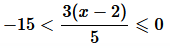Solution :
Given:

⇒  −12x−12<30−12

⇒ −75<3(x−2)⩽0

⇒ −25<x−2⩽0

⇒ −25+2<x⩽2

⇒ −23<x⩽2

As a result, the set of solutions for the given inequality is (-23, 2]

Question5.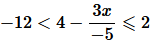Solution :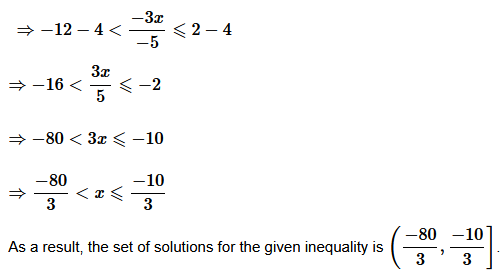Question6.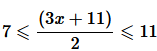Solution :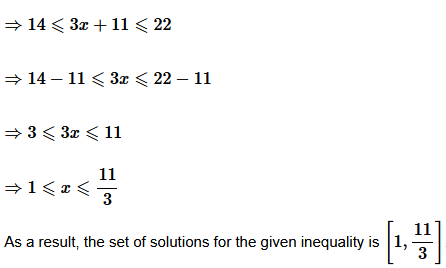Question7. Solve the inequalities and represent the solution graphically on number line: 5x + 1 > –24, 5x – 1 < 24

Solution :
5x + 1 > –24

⇒ 5x > –25

⇒ x > –5 … (1)

5x – 1 < 24

⇒ 5x < 25

⇒ x < 5 … (2)

From (1) and (2), it can be concluded that the solution set for the given system of inequalities is (–5, 5). The solution of the given system of inequalities can be represented on number line as

Question8. Solve the inequalities and represent the solution graphically on number line: 2(x – 1) < x + 5, 3(x + 2) > 2 – x

Solution :
2(x – 1) < x + 5

⇒ 2x – 2 < x + 5

⇒ 2x – x < 5 + 2

⇒ x < 7 … (1)

3(x + 2) > 2 – x

⇒ 3x + 6 > 2 – x

⇒ 3x + x > 2 – 6

⇒ 4x > – 4

⇒ x > – 1 … (2)

From (1) and (2), it can be concluded that the solution set for the given system of inequalities is (–1, 7). The solution of the given system of inequalities can be represented on number line as

Question9. Solve the following inequalities and represent the solution graphically on number line:

3x – 7 > 2(x – 6), 6 – x > 11 – 2x

Solution :
3x – 7 > 2(x – 6)

⇒ 3x – 7 > 2x – 12

⇒ 3x – 2x > – 12 + 7

⇒ x > –5 … (1)

6 – x > 11 – 2x

⇒ –x + 2x > 11 – 6

⇒ x > 5 … (2)

From (1) and (2), it can be concluded that the solution set for the given system of inequalities is(5, ). The solution of the given system of inequalities can be represented on number line

Question10. Solve the inequalities and represent the solution graphically on number line: 5 (2x – 7) – 3 (2x + 3) ≤ 0 , 2x + 19 ≤ 6x + 47 .

Solution :
5(2x – 7) – 3(2x + 3) ≤ 0

⇒ 10x – 35 – 6x – 9 ≤ 0

⇒ 4x – 44 ≤ 0

⇒ 4x ≤ 44

⇒ x ≤ 11 … (1)

2x + 19 ≤ 6x + 47

⇒ 19 – 47 ≤ 6x – 2x

⇒ –28 ≤ 4x

⇒ –7 ≤ x … (2)

From (1) and (2), it can be concluded that the solution set for the given system of inequalities is [–7, 11].

The solution of the given system of inequalities can be represented on number line as

Question11. A solution is to be kept between 68°F and 77°F. What is the range of temperature in degree Celsius (C) if the Celsius / Fahrenheit (F) convension formula is given by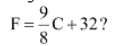?

Solution :
Given 68°F and 77°F.

Question12. A solution of 8% boric acid is to be diluted by adding a 2% boric acid solution to it. The resulting mixture is to be more than 4% but less than 6% boric acid. If we have 640 litres of the 8% solution, how many litres of the 2% solution will have to be added?

Solution :
Let x litres of 2% boric acid solution is required to be added.

Then, total mixture = (x + 640) litres

This resulting mixture is to be more than 4% but less than 6% boric acid.

∴2%x + 8% of 640 > 4% of (x + 640)

And, 2% x + 8% of 640 < 6% of (x + 640)

2%x + 8% of 640 > 4% of (x + 640)

⇒ 2x + 5120 > 4x + 2560

⇒ 5120 – 2560 > 4x – 2x

⇒ 5120 – 2560 > 2x

⇒ 2560 > 2x

⇒ 1280 > x

2% x + 8% of 640 < 6% of (x + 640)

⇒ 2x + 5120 < 6x + 3840

⇒ 5120 – 3840 < 6x – 2x

⇒ 1280 < 4x

⇒ 320 < x

∴320 < x < 1280

Thus, the number of litres of 2% of boric acid solution that is to be added will have to be more than 320 litres but less than 1280 litres.

Question13. How many liters of water will have to be added to 1125 liters of the 45% solution of acid so that the resulting mixture will contain more than 25% but less than 30% acid content?

Solution :
Allow for the addition of x litres of water. The entire mixture is then calculated = (x + 1125) litres It is clear that the amount of acid in the final mixture is excessive. 45% of 1125 litres. The resulting mixture will have a higher concentration of 25% but less than 30% acid content.

Therefore, minimum 562.5 liters and maximum 900 liters of water need to be added.

Question14. IQ of a person is given by the formula IQ = Where MA is mental age and CA is chronological age. If 80 ≤ IQ ≤ 140 for a group of 12 years old children, find the range of their mental age.

Solution :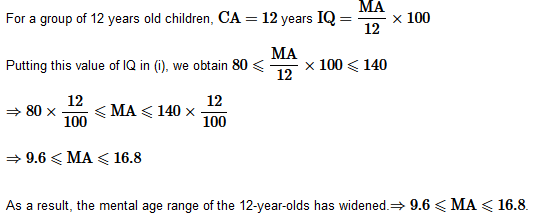Get 30% off your first purchase!

X
error: Content is protected !!
Scroll to Top